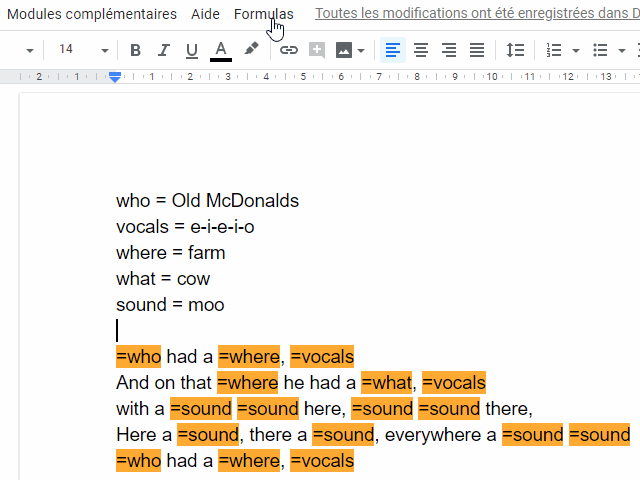2019/02/13 — Mikaël Mayer.
Have you ever wished you could synchronize content within one Google doc? Do you need temporary placeholders that you will replace later? Do you want to generate text? Do you miss spreadsheets in docs?

If you answered yes to one of these questions, this Google docs add-on this for you. It enables you to define names (a.k.a. variables) anywhere in the document, and to insert computations that produce rich text anywhere in the document.

## Define names

You can define a name anywhere in your document. It has to start with a word, the sign equal '=' and a non-empty space behind it. If there is an opening parenthesis, the content will be evaluated as JavaScript. If there is no opening parenthesis, the remaining until the end of the newline is treated as raw text. Definitions can depend on previous definitions.
The following are all valid definitions of names:
version = 1.0 beta
name = ("MySoft" + version)
citations = ({ mayer18: "https://dl.acm.org/citation.cfm?id=3276497", chugh16: "https://dl.acm.org/citation.cfm?id=2908103" })
cite = (function (name) { return typeof citations[name] !== "undefined" ? ["[" + name + "]", {link: citations[name]}] : "[" + name + "???]"; })

## Write formulas in your document

Anywhere in the document, write = followed by either
• a name
• a name followed by a JavaScript expression in parentheses
• a JavaScript expression inside parentheses or square brackets.
If, right after the first opening '(', there is a comment such as /*name=*/, name will be defined with the result of the expression.
For example, in a document, you would write raw
=name is out! =[name, {bold: true}] is a n=(function() { var x = ""; var i = 10; while(i-- > 0) x = x + "i"; return x} ())ce =(/*s=*/"software") based on bidirectional evaluation =cite("mayer18") that goes beyond the original ideas of prodirect manipulation =s =cite("chugh16")

## Render formulas

Open the menu "Formulas" and click on "Evaluate formulas". It replaces all formulas by their values. If you selected some text, only formulas in the selection would be evaluated. The document above would be rendered as:
MySoft 1.0 beta is out! MySoft 1.0 beta is a niiiiiiiiiice software based on bidirectional evaluation [mayer18] that goes beyond the original ideas of prodirect manipulation software [chugh16]
Formulas used in the document should either return raw text, or a 2-element array where the first element is the text, and the second is a Javascript object containing styling material. If the method `setThing` exists in this page, then `thing : value` is a valid style attribute. Here is a list of supported attributes and shortcuts
• `backgroundColor` (or `background`): a string representing a color like `"#FF00FF"`.
• `bold`: boolean (`true` or `false`).
• `fontFamily`: string representing a valid font name.
• `fontSize`: integer representing a valid font size.
• `foregroundColor` (or `color`): a string representing a color like `"#FF00FF"`.
• `italic`: boolean (`true` or `false`).
• `linkUrl` (or `link`): string representing an URL.
• `strikeThrough` (or `strike`): boolean (`true` or `false`).
• `textAlignment` (or `align`): either `"normal"`, `"superscript"` or `"subscript"`
• `underline`: boolean (`true` or `false`).

## Visualize where formulas are being used

To make sure a field is computed and not just text, open "Formulas" and then click on "Highlight computed fields". It highlights all places where the text is computed. If you selected some text, only computed text in the selection would be highlighted. The document above would be transformed to:
MySoft 1.0 beta is out! MySoft 1.0 beta is a niiiiiiiiiice software based on bidirectional evaluation [mayer18] that goes beyond the original ideas of prodirect manipulation software [chugh16]
You can hide these highlights by opening "Formulas" and click on "Remove highlights". If you selected some text, only highlights in the selection would be removed.

## Reveal formulas

It can be useful to change formulas. To do so, open "Formulas" and click on "Reveal formulas". It will replace all computed fields by their respective formulas and highlight them in orange. If you selected a portion of the text, only the formulas in the selection would be revealed. You can then edit any formula:
=name is out! =name is a n=(function() { var x = ""; var i = 10; while(i-- > 0) x = x + "i"; return x} ())ce =(/*s=*/"software") based on bidirectional evaluation =cite("mayer18") that goes beyond the original ideas of prodirect manipulation =s =cite("chugh16")
Once you're done, click on "Formulas" and then "Evaluate formulas" to replace the formulas by their values.
=name is out! MySoft 1.0 is a niiiiiiiiiice software based on bidirectional evaluation [mayer18] that goes beyond the original ideas of prodirect manipulation software [chugh16]

## Availability

• Modify a formula or a name's value by modifying its output anywhere. It'll work for text variables only soon: